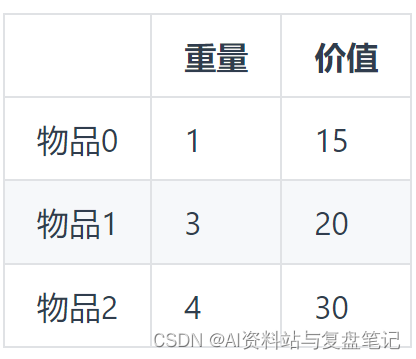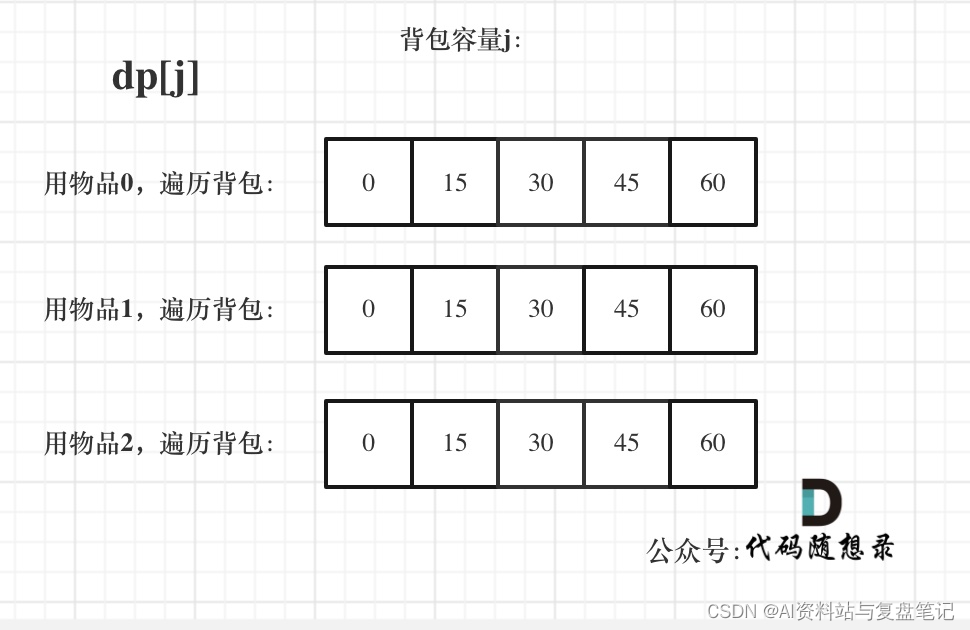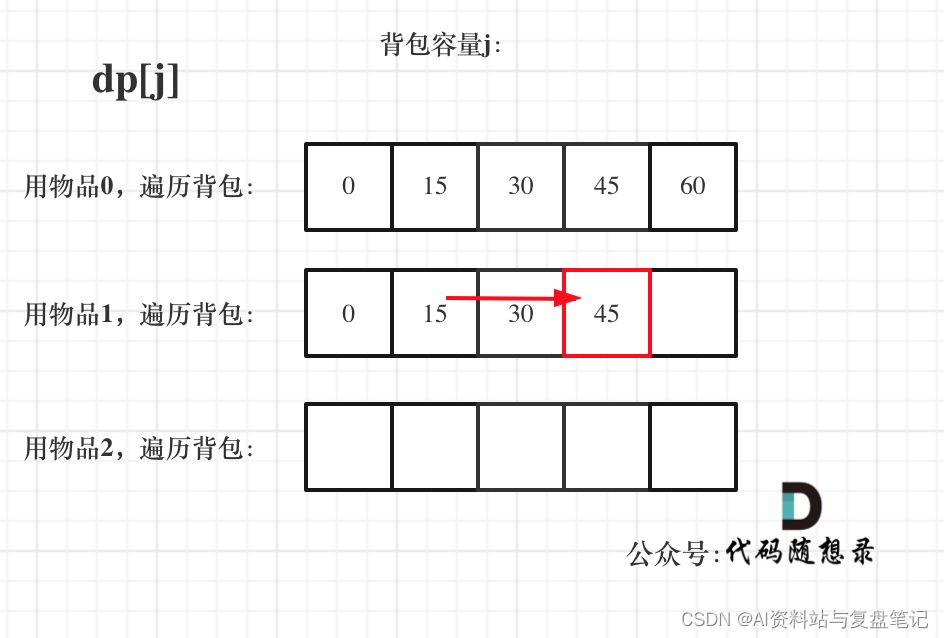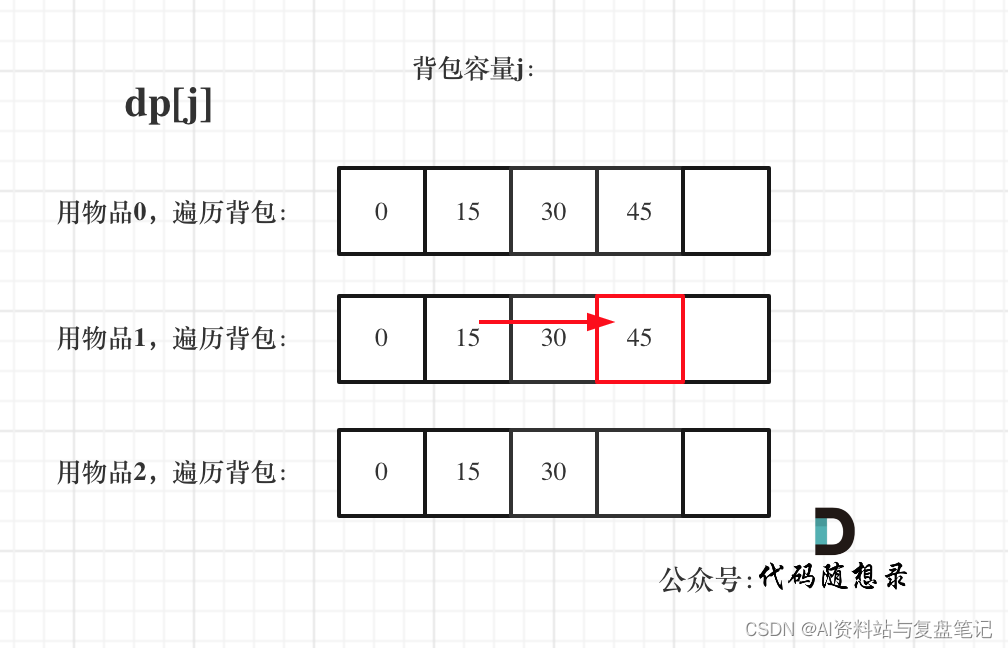# 题目：纯完全背包问题### 本题重难点

0-1背包和完全背包唯一不同就是体现在遍历顺序上，所以本文就不去做动规五部曲了，我们直接针对遍历顺序经行分析！

``````for(int i = 0; i < weight.size(); i++) { // 先遍历物品
for(int j = bagWeight; j >= weight[i]; j--) { // 再遍历背包容量
dp[j] = max(dp[j], dp[j - weight[i]] + value[i]);
}
}
``````

``````// 先遍历物品，再遍历背包
for(int i = 0; i < weight.size(); i++) { // 遍历物品
for(int j = weight[i]; j <= bagWeight ; j++) { // 遍历背包容量
dp[j] = max(dp[j], dp[j - weight[i]] + value[i]);

}
}
``````

dp状态图如下：``````// 先遍历背包，再遍历物品
for(int j = 0; j <= bagWeight; j++) { // 遍历背包容量
for(int i = 0; i < weight.size(); i++) { // 遍历物品
if (j - weight[i] >= 0) dp[j] = max(dp[j], dp[j - weight[i]] + value[i]);
}
cout << endl;
}
``````

# 总结

1. 确定dp数组（dp table）以及下标的含义
2. 确定递推公式
3. dp数组如何初始化
4. 确定遍历顺序
5. 举例推导dp数组
以上的讲解可以开发一道面试题目（毕竟力扣上没原题）。

(关注后可以免费获得本人在csdn发布的资源源码)